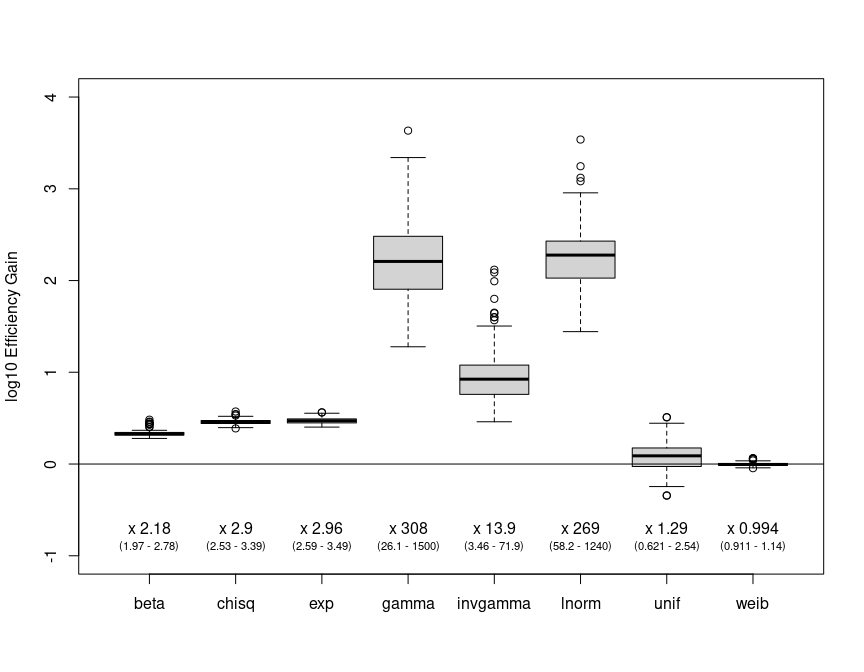# A quick guide to nimbleNoBounds

This vignette assumes the reader is already familiar with using NIMBLE to perform Markov chain Monte Carlo (MCMC).

The principal motivation of this package is that bounds on prior distributions can impair MCMC performance when posterior distributions are located close to those bounds. In particular, when using Metropolis-Hastings, proposals made beyond the known bounds of a distribution will inflate rejection rates. Moreover, in adaptive Metropolis-Hastings, such increased rejection rates will lead to shrinking the Metropolis-Hastings kernel, thereby increasing the auto-correlation in the accepted samples and reducing the effective sample size. The result can therefore be reduced MCMC efficiency.

This package offers a simple solution to avoid this scenario. Common bounded probability distributions (beta, uniform, exponential, gamma, chi-squared, half-flat, inverse-gamma, log-normal, Weibull) are transformed, via log or logit transformations, to the unbounded real line. The change of variables technique is used to obtain the transformed distributions. The resulting distributions are provided here for use in NIMBLE models.

In this vignette we explore the potential efficiency gains with a simple toy example.

# Distributions included in nimbleNoBounds

The following transformed distributions are available.

Function Transform Distribution Corresponding R function
dLogChisq Log Chi-squared dchisq
dLogExp Log Exponential dexp
dLogGamma Log Gamma dgamma
dLogHalfflat Log Half-flat dhalfflat
dLogInvgamma Log Inverse-gamma dinvgamma
dLogLnorm Log Log-normal dlnorm
dLogWeib Log Weibull dweib
dLogitBeta Logit Beta dbeta
dLogitUnif Logit Uniform dunif

Note: the naming convention is d[Transform][Distribution]. Only d and r functions have been provided. I do not plan to add p or q functions, but am open to collaboration if somebody else is motivated to add them.

# Toy example

The following toy problem compares sampling of bounded and unbounded distributions.

First, we create NIMBLE models with both classical bounded distributions, and with unbounded transformed equivalents.

library(nimbleNoBounds) # Also loads nimble
library(coda)           # For MCMC diagnostics

## Model with bounded priors
boundedDistributionsModel <- nimbleCode({
b  ~ dbeta(b1, b2)
c  ~ dchisq(c1)
e  ~ dexp(e1)
g  ~ dgamma(g1, g2)
ig ~ dinvgamma(i1, i2)
l  ~ dlnorm(l1, l2)
u  ~ dunif(u1, u2)
w  ~ dweib(w1, w2)
})

## Model with unbounded priors
unboundedDistributionsModel <- nimbleCode({
## Priors transformed to real line
tb  ~ dLogitBeta(b1, b2)
tc  ~ dLogChisq(c1)
te  ~ dLogExp(e1)
tg  ~ dLogGamma(g1, g2)
tl  ~ dLogLnorm(l1, l2)
tu  ~ dLogitUnif(u1, u2)
tw  ~ dLogWeib(w1, w2)
## Back-transformations
b  <- ilogit(tb)
c  <- exp(tc)
e  <- exp(te)
g  <- exp(tg)
ig <- exp(tig) ## Currently doesn't compile if a node is called "i"
l  <- exp(tl)
u  <- (u2*exp(tu)+u1)/(u2-u1)
w  <- exp(tw)
})

## List of (fixed) parameters
const = list(
b1=1   , b2=11,     ## Beta
c1=2   ,            ## Chi-squared
i1=2.5 , i2=0.01,   ## Inverse-gamma
u1=-6  , u2=66,     ## Uniform
e1=0.1 ,            ## Exponential
g1=0.1 , g2=10,     ## Gamma
l1=-3  , l2=0.1,    ## Log-normal
w1=2   , w2=2       ## Weibull
)

## Build and compile models
rBounded   <- nimbleModel(boundedDistributionsModel, constants=const)
rUnbounded <- nimbleModel(unboundedDistributionsModel, constants=const)
cBounded   <- compileNimble(rBounded)
cUnbounded <- compileNimble(rUnbounded)

## Lists of nodes
stochNodesB   = rBounded$getNodeNames(stochOnly=TRUE) stochNodesU = rUnbounded$getNodeNames(stochOnly=TRUE)
monitorNodes  = stochNodesB
distributions = substring(rBounded$getDistribution(monitorNodes), 2, 99) Now we perform MCMC using NIMBLE’S univariate Metropolis-Hastings sampler. We use B to indicate bounded and U to indicate unbounded. The aim is to calculate the efficiency gain when sampling each distribution on the real line. This is done within a loop so that between-run variation can be accounted for. Efficiency is calculated as the ratio of effective sample size divided by time spent executing a given sampler. The efficiency gain is then calculated as the ratio (unbounded over bounded) of the efficiencies. ## Configure an MCMC for each model configureMcmcB = configureMCMC(cBounded, monitors=monitorNodes) configureMcmcU = configureMCMC(cUnbounded, monitors=monitorNodes) ## Remove posterior predictive samplers & replace with univariate Metropolis-Hastings configureMcmcB$removeSamplers()
configureMcmcU$removeSamplers() for (nd in stochNodesB) configureMcmcB$addSampler(nd)
for (nd in stochNodesU) configureMcmcU$addSampler(nd) print(configureMcmcB) print(configureMcmcU) ## Build & compile the MCMCs rMcmcB <- buildMCMC(configureMcmcB) rMcmcU <- buildMCMC(configureMcmcU) cMcmcB <- compileNimble(rMcmcB) cMcmcU <- compileNimble(rMcmcU) ## The following loop takes approximately 10 minutes. ## You can optionally skip running the loop and load the data object efficiencyGain from the package. ## Or simply just reduce nSamples and nReps. runLoop = FALSE if (runLoop) { nSamples = 1E5 nReps = 111 efficiencyGain = matrix(0, ncol=length(monitorNodes), nrow=nReps) for (ii in 1:nReps) { print(ii) ## Run MCMC cMcmcB$run(niter=nSamples, time = TRUE)
cMcmcU$run(niter=nSamples, time = TRUE) ## Extract samples samplesB = as.matrix(cMcmcB$mvSamples)
samplesU = as.matrix(cMcmcU$mvSamples) ## Calculate effective sample size (effB = effectiveSize(samplesB)) (effU = effectiveSize(samplesU)) ## Time spent in each sampler timeB = cMcmcB$getTimes()
timeU = cMcmcU\$getTimes()
## Calculate sampling efficiency
(efficiencyB = effB / timeB)
(efficiencyU = effU / timeU)
## Calculate efficiencyGain
(efficiencyGain[ii,] = efficiencyU / efficiencyB)
}
colnames(efficiencyGain) = distributions
## write.table(efficiencyGain, file=here::here("nimbleNoBounds/data/efficiencyGain.csv"), sep=";", row.names = FALSE)
}

Finally, some box-plots of the efficiency gain per distribution, annotated with the with mean and 95% CIs.

data(efficiencyGain, package="nimbleNoBounds")

boxplot(log10(efficiencyGain), ylab="log10 Efficiency Gain", ylim=c(-1, 4))
abline(h=0)
for (ii in 1:length(distributions)) {
text(ii, -0.7, paste("x", signif(mean(efficiencyGain[,distributions[ii]]), 3)))
text(ii, -0.9, paste0("(", paste(signif(quantile(efficiencyGain[,distributions[ii]], c(0.025, 0.975)), 3), collapse=" - "), ")"), cex=0.7)
}These results show that:

1. large efficiency gains can be obtained from sampling on the real line.
2. the size of the efficiency gain depends on the target distribution being sampled.
3. computational overhead arising from the transformations is negligible compared to potential efficiency gains from sampling on the unbounded real line.